# [转载]基于事件通信的轻量级MVP框架实现，附源码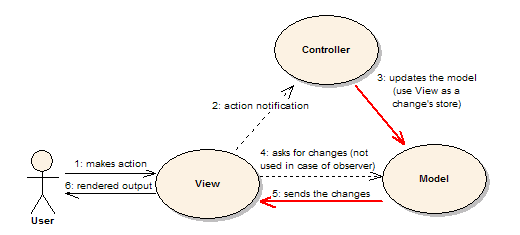Figure 1 主动MVC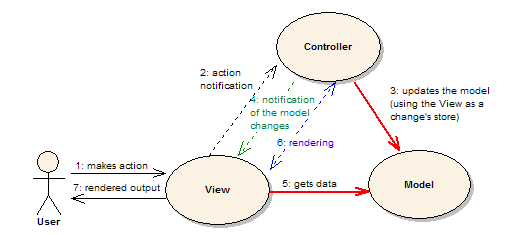Figure 2 被动MVC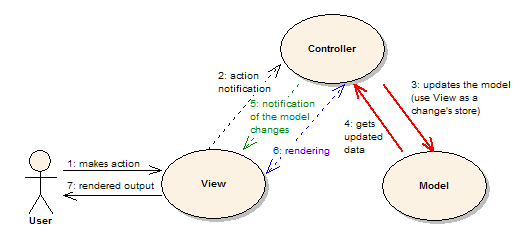Figure 3 MVP模式

MVP具体到.NET中来，在其中使用的Observer模式自然可以用事件实现。笔者认为虽然一个Presenter有可能会存在需要多 个视图以及多个Model的情况，但是大多情况下，一个Presenter仅仅关注一个Model。对于View，情况则比较复杂，通常为了将某一类 Model的信息显示出来，我们会为其专门定制一个View，但是，我们还需要输出许多信息，看上去我们是需要向其他的View发送信息。但是，如果我们 为每一个View都做一个Presenter，一个Model的话，我们只需要在当前Presenter引用那个View所对应的Model，发送相应信 息就好了。所以，笔者的原则就是，不要让更新不属于当前Presenter的View，而是通过该Presenter引用Model实现其他信息的输出。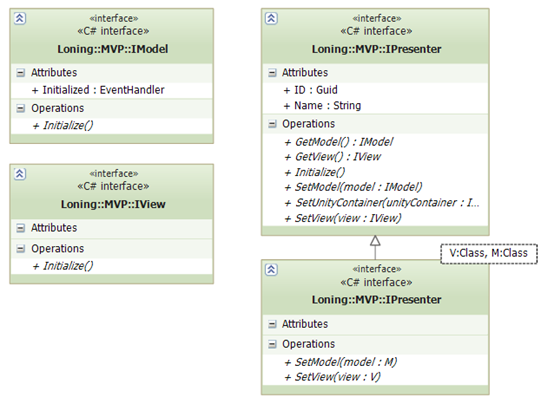Figure 4 框架接口

 `01` `public` `interface` `IView`
 `02` ` ``{`
 `03` ` ``void` `Initialize();`
 `04` ` ``}`
 `05` ` ``public` `interface` `IModel`
 `06` ` ``{`
 `07` ` ``void` `Initialize();`
 `08` ` ``event` `EventHandler Initialized;`
 `09` ` ``}`
 `10` ` ``public` `interface` `IPresenter`
 `11` ` ``{`
 `12` ` ``void` `SetView(IView view);`
 `13` ` ``void` `SetModel(IModel model);`
 `14` ` ``void` `SetUnityContainer(IUnityContainer unityContainer);`
 `15` ` ``void` `Initialize();`
 `16` ` ``string` `Name { ``get``; }`
 `17` ` ``Guid ID { ``get``; }`
 `18` ` ``IView GetView();`
 `19` ` ``IModel GetModel();`
 `20` ` ``}`
 `21` ` ``public` `interface` `IPresenter : IPresenter`
 `22` ` ``where V : IView`
 `23` ` ``where M : IModel`
 `24` ` ``{`
 `25` ` ``void` `SetView(V view);`
 `26` ` ``void` `SetModel(M model);`
 `27` ` ``}`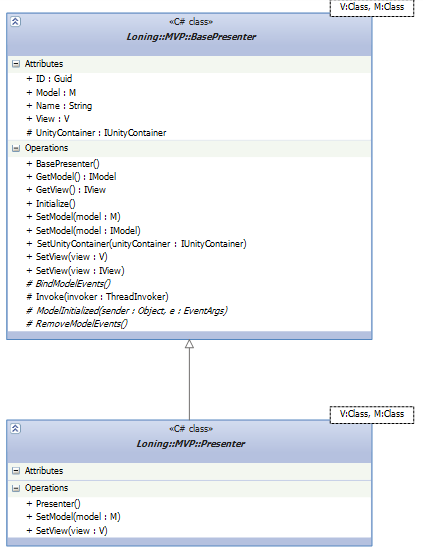Figure 5 Presenter基类

 `1` `public` `interface` `IHelloMvpModel : IModel`
 `2` ` ``{`
 `3` ` ``void` `Plus();`
 `4` ` ``int` `GetNumber { ``get``; }`
 `5` ` ``event` `EventHandler NumberChanged;`
 `6` ` ``}`

 `1` `public` `interface` `IHelloMvpView : IView`
 `2` ` ``{`
 `3` ` ``void` `SetNumber(``int` `number);`
 `4` ` ``event` `EventHandler ChangeNumberRequested;`
 `5` ` ``}`

 `01` `static` `void` `Main()`
 `02` ` ``{`
 `03` ` ``Application.EnableVisualStyles();`
 `04` ` ``Application.SetCompatibleTextRenderingDefault(``false``);`
 `05`
 `06` ` ``IUnityContainer container = ``new` `UnityContainer();`
 `07` ` ``container.RegisterInstance(container);`
 `08` ` ``container.RegisterType();`
 `09` ` ``container.RegisterType();`
 `10`
 `11` ` ``var p = container.Resolve();`
 `12` ` ``p.Initialize();`
 `13` ` ``Application.Run((Form) p.View);`
 `14` ` ``}`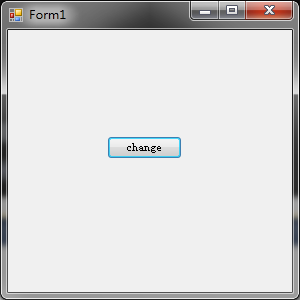Figure 6 运行截图Figure 7 点击按钮后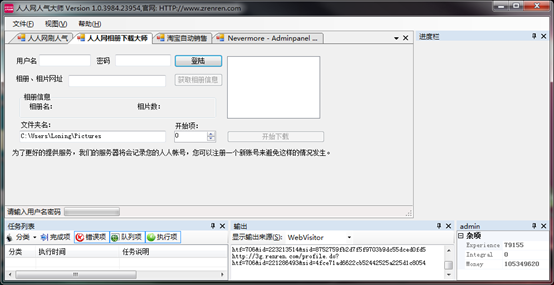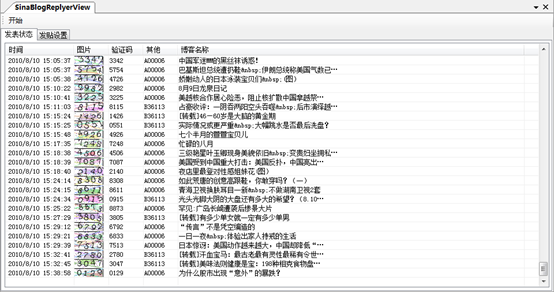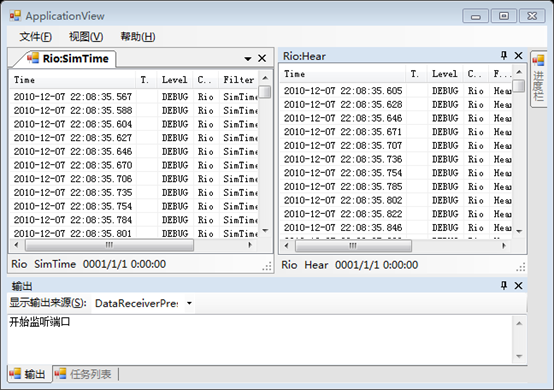### 觉得文章有用就打赏一下文章作者

#### 支付宝扫一扫打赏#### 微信扫一扫打赏• QQ咨询
• 回顶